# Design Broadband Matching Networks for Amplifier

This example shows how to design broadband matching networks for a low noise amplifier (LNA) with ideal and real-world lumped LC elements. The real-world lumped LC elements are obtained from the Modelithics SELECT+ Library™. The LNA is designed to the target gain and noise figure specifications over a specified bandwidth. A direct-search based approach is used to arrive at the optimum element values in the input and output matching networks.

In an RF receiver front end, the LNA is commonly found immediately after the antenna or after the first bandpass filter that follows the antenna. Its position in the receiver chain ensures that it deals with weak signals that have significant noise content. As a result the LNA has to not only provide amplification to such signals but also minimize its own noise footprint on the amplified signal.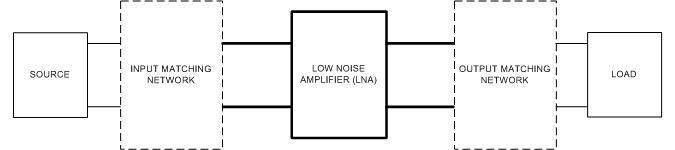Figure 1: Impedance matching of an amplifier

### Set Design Parameters

The design specifications are as follows.

• Amplifier is an LNA amplifier

• Center Frequency = 250 MHz

• Bandwidth = 100 MHz

• Transducer Gain greater than or equal to 10 dB

• Noise Figure less than or equal to 2.0 dB

• Operating between 50-Ohm terminations

### Specify Design Parameters

You are building the matching network for an LNA with a bandpass response, so specify the bandwidth of match, center frequency, gain, and noise figure targets.

```BW = 100e6; % Bandwidth of matching network (Hz) fc = 250e6; % Center frequency (Hz) Gt_target = 10; % Transducer gain target (dB) NFtarget = 2; % Max noise figure target (dB)```

Specify the source impedance, reference impedance, and the load impedance.

```Zs = 50; % Source impedance (Ohm) Z0 = 50; % Reference impedance (Ohm) Zl = 50; % Load impedance (Ohm)```

### Create Amplifier Object and Perform Analysis

Create an `amplifier` object from `lnadata.s2p`.

`Mismatched_Amp = amplifier(FileName='lnadata.s2p');`

Define the number of frequency points to use for analysis and set up the frequency vector.

```Npts = 32; % No. of analysis frequency points fLower = fc - (BW/2); % Lower band edge fUpper = fc + (BW/2); % Upper band edge freq = linspace(fLower,fUpper,Npts); % Frequency array for analysis```

Perform frequency domain analysis on the mismatched amplifier.

```sparam = sparameters(Mismatched_Amp,freq); bMismatched = rfbudget(Mismatched_Amp,freq',-30,BW); [K,~,~,Delta] = stabilityk(sparam); Ga = powergain(sparam,'Ga');```

### Examine Stability, Power Gain, and Noise Figure

Plot the LNA stability parameters and examine stability, power gain and noise figure.

```figure plot(freq*1e-6,abs(Delta)) hold on plot(freq*1e-6,K) legend('Delta','K','Location','best') title('Device stability parameters') xlabel('Frequency [MHz]') ylabel('Magnitude') grid on hold off```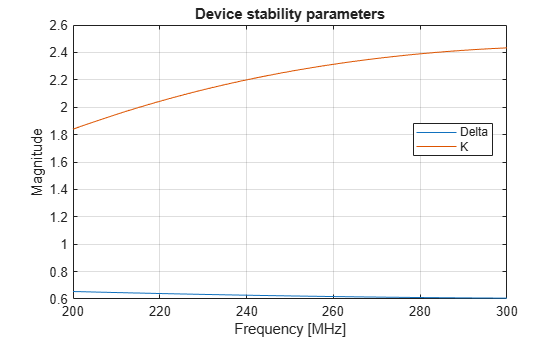As the plot shows, $K>1$ and $\Delta <1$ for all frequencies in the bandwidth of interest. This means that the device is unconditionally stable. It is also important to view the power gain and noise figure behavior across the same bandwidth. Together with the stability information this data allows you to determine if the gain and noise figure targets can be met.

```figure plot(freq*1e-6,10*log10(Ga)) hold on plot(freq*1e-6,bMismatched.TransducerGain) legend('Ga','Gt','Location','east') xlabel('Frequency [MHz]') ylabel('Magnitude [dB]') hold off grid on```This plot, shows the power gain across the 100-MHz bandwidth. It indicates that the transducer gain varies linearly between 5.5 dB to about 3.1 dB and achieves only 4.3 dB at band center. It also suggests there is sufficient headroom between the transducer gain `Gt` and the available gain Ga to achieve our target `Gt` of 10 dB.

```figure plot(Mismatched_Amp.NoiseData.Frequencies*1e-6,Mismatched_Amp.NoiseData.Fmin) hold on plot(freq*1e-6,bMismatched.NF) hold off axis([200 300 0 2]) legend('Fmin','NF','Location','NorthEast') xlabel('Frequency [MHz]') ylabel('Magnitude [dB]') grid on```This plot shows the variation of the noise figure with frequency. The unmatched amplifier clearly meets the target noise figure requirement. However this would change once the input and output matching networks are included. Most likely, the noise figure of the LNA would exceed the requirement.

The region of operation is between 200 300 MHz. Therefore, choose a bandpass topology for the matching networks which is shown here.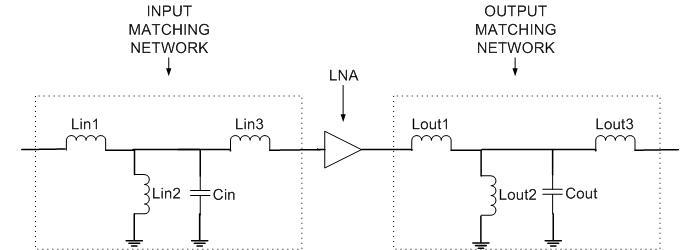Figure 2: Matching network topology

The topology chosen, as seen in Figure 2, is a direct-coupled prototype bandpass network of parallel resonator type with top coupling , that is initially tuned to the geometric mean frequency with respect to the bandwidth of operation.

```N = 3; % Order of input/output matching network wU = 2*pi*fUpper; % Upper band edge wL = 2*pi*fLower; % Lower band edge w0 = sqrt(wL*wU); % Geometric mean```

For the initial design all the inductors are assigned the same value on the basis of the first series inductor. As mentioned in , choose the prototype value to be unity and use standard impedance and frequency transformations to obtain denormalized values . The value for the capacitor in the parallel trap is set using this inductor value to make it resonate at the geometric mean frequency. Note that there are many ways of designing the initial matching network. This example shows one possible approach.

```LvaluesIn = (Zs/(wU-wL))*ones(N,1); % Series and shunt L's [H] CvaluesIn = 1 / ( (w0^2)*LvaluesIn(2)); % Shunt C [F] LvaluesOut = LvaluesIn; CvaluesOut = CvaluesIn;```

### Build Matching Networks

Build each branch of the matching network and then define the circuit as a two-port network. This example uses identical input and output matching networks.

```InputMatchingNW = circuit; add(InputMatchingNW,[1 2],inductor(LvaluesIn(1))); add(InputMatchingNW,[2 0],inductor(LvaluesIn(2))); add(InputMatchingNW,[2 0],capacitor(CvaluesIn)); add(InputMatchingNW,[2 3],inductor(LvaluesIn(3))); setports(InputMatchingNW,[1 0],[3 0]); OutputMatchingNW = circuit; add(OutputMatchingNW,[1 2],inductor(LvaluesOut(1))); add(OutputMatchingNW,[2 0],inductor(LvaluesOut(2))); add(OutputMatchingNW,[2 0],capacitor(CvaluesOut)); add(OutputMatchingNW,[2 3],inductor(LvaluesOut(3))); setports(OutputMatchingNW,[1 0],[3 0]);```

### Optimize Input & Output Matching Network

There are several points to consider prior to the optimization.

• Objective function: The objective function can be built in different ways depending on the problem at hand. For this example, the objective function is shown in the file below.

• Choice of cost function: The cost function is the function you would like to minimize (maximize) to achieve near optimal performance. There could be several ways to choose the cost function. For this example you have two requirements to satisfy simultaneously, i.e. gain and noise figure. To create the cost function you first, find the difference, between the most current optimized network and the target value for each requirement at each frequency. The cost function is the L2-norm of the vector of gain and noise figure error values.

• Optimization variables: In this case it is a vector of values, for the specific elements to optimize in the matching network.

• Optimization method: A direct search based technique, the MATLAB® function `fminsearch`, is used in this example to perform the optimization.

• Number of iterations/function evaluations: Set the maximum no. of iterations and function evaluations to perform, so as to tradeoff between speed and quality of match.

• Tolerance value: Specify the variation in objective function value at which the optimization process should terminate.

The objective function used during the optimization process by `fminsearch` is shown here.

`type('broadband_match_amplifier_objective_function.m')`
```function output = broadband_match_amplifier_objective_function(AMP,inMNW,outMNW,LC_Optim,freq,Gt_target,NF) %BROADBAND_MATCH_AMPLIFIER_OBJECTIVE_FUNCTION is the objective function. % OUTPUT = BROADBAND_MATCH_AMPLIFIER_OBJECTIVE_FUNCTION(AMP,INMNW,OUTMNW,LC_OPTIM,FREQ,GT_TARGET,NF) % returns the current value of the objective function stored in OUTPUT % evaluated after updating the element values in the object, inMNW and % outMNW. The inductor and capacitor values are stored in the variable % LC_OPTIM. % % BROADBAND_MATCH_AMPLIFIER_OBJECTIVE_FUNCTION is an objective function of RF Toolbox demo: % Designing Broadband Matching Networks (Part II: Amplifier) % Copyright 2023 The MathWorks, Inc. % Ensure positive element values if any(LC_Optim<=0) output = inf; return; end % Update matching network elements - The object inMNW and outMNW have % several properties among which the cell array 'Elements' consists of all % LC elements in the circuit. inMNW.Elements(1).Inductance = LC_Optim(1); inMNW.Elements(2).Inductance = LC_Optim(2); inMNW.Elements(4).Inductance = LC_Optim(3); inMNW.Elements(3).Capacitance = LC_Optim(4); outMNW.Elements(1).Inductance = LC_Optim(5); outMNW.Elements(2).Inductance = LC_Optim(6); outMNW.Elements(4).Inductance = LC_Optim(7); outMNW.Elements(3).Capacitance = LC_Optim(8); % Perform analysis on tuned matching network Npts = length(freq); BW = max(freq) - min(freq); np1 = nport(sparameters(inMNW,freq)); np2 = nport(sparameters(outMNW,freq)); b = rfbudget([np1 clone(AMP) np2],freq',-30,BW); % Calculate transducer power gains and noise figures of the amplifier Gt = b.TransducerGain(:,end); NF_amp = b.NF(:,end); % Calculate target gain and noise figure error errGt = (Gt - Gt_target); errNF = (NF_amp - NF); % Check to see if gain and noise figure target are achieved by specifying % bounds for variation. deltaG = 0.40; deltaNF = -0.05; errGt(abs(errGt)<=deltaG) = 0; errNF(errNF<deltaNF) = 0; % Cost function err_vec = [errGt;errNF]; output = norm((err_vec),2); % Animate Gmax = (Gt_target + deltaG).*ones(1,Npts); Gmin = (Gt_target - deltaG).*ones(1,Npts); plot(freq*1e-6,Gt) hold on plot(freq*1e-6,NF_amp) plot(freq.*1e-6,Gmax,'r-*') plot(freq.*1e-6,Gmin,'r-*') legend('G_t','NF','Gain bounds','Location','East'); xlabel('Freq [MHz]') ylabel('Magnitude [dB]') axis([freq(1)*1e-6 freq(end)*1e-6 0 Gt_target+2]); hold off drawnow; ```

The optimization variables are all the elements (inductors and capacitors) of the input and output matching networks.

```nIter = 125; % Max No of Iterations options = optimset('Display','iter','TolFun',1e-2,'MaxIter',nIter); % Set options structure LC_Optimized = [LvaluesIn;CvaluesIn;LvaluesOut;CvaluesOut]; LC_Optimized = fminsearch(@(LC_Optimized) broadband_match_amplifier_objective_function(Mismatched_Amp,InputMatchingNW,... OutputMatchingNW,LC_Optimized,freq,Gt_target,NFtarget),LC_Optimized,options);```
``` Iteration Func-count f(x) Procedure 0 1 30.4869 1 9 28.3549 initial simplex 2 11 25.5302 expand 3 12 25.5302 reflect 4 13 25.5302 reflect 5 14 25.5302 reflect 6 16 22.8228 expand 7 17 22.8228 reflect 8 19 19.0289 expand 9 20 19.0289 reflect 10 21 19.0289 reflect 11 22 19.0289 reflect 12 24 14.8785 expand 13 25 14.8785 reflect 14 27 10.721 expand 15 28 10.721 reflect 16 29 10.721 reflect 17 31 9.84796 expand 18 32 9.84796 reflect 19 33 9.84796 reflect 20 34 9.84796 reflect 21 35 9.84796 reflect 22 37 9.84796 contract outside 23 39 9.84796 contract outside 24 41 9.84796 contract inside 25 43 9.64666 reflect 26 45 9.64666 contract inside 27 46 9.64666 reflect 28 48 9.64666 contract inside 29 49 9.64666 reflect 30 51 9.64666 contract inside 31 53 7.9372 expand 32 55 7.9372 contract outside 33 56 7.9372 reflect 34 57 7.9372 reflect 35 58 7.9372 reflect 36 59 7.9372 reflect 37 60 7.9372 reflect 38 62 5.98211 expand 39 63 5.98211 reflect 40 64 5.98211 reflect 41 65 5.98211 reflect 42 66 5.98211 reflect 43 68 4.31973 expand 44 70 4.31973 contract inside 45 71 4.31973 reflect 46 72 4.31973 reflect 47 73 4.31973 reflect 48 74 4.31973 reflect 49 75 4.31973 reflect 50 77 2.83135 expand 51 79 1.17624 expand 52 80 1.17624 reflect 53 81 1.17624 reflect 54 82 1.17624 reflect 55 84 0.691645 reflect 56 85 0.691645 reflect 57 86 0.691645 reflect 58 88 0.691645 contract inside 59 90 0.691645 contract outside 60 91 0.691645 reflect 61 93 0.691645 contract inside 62 95 0.691645 contract inside 63 96 0.691645 reflect 64 97 0.691645 reflect 65 98 0.691645 reflect 66 100 0.691645 contract inside 67 102 0.691645 contract outside 68 103 0.691645 reflect 69 105 0.691645 contract inside 70 107 0.497434 reflect 71 109 0.497434 contract inside 72 111 0.497434 contract inside 73 112 0.497434 reflect 74 114 0.497434 contract inside 75 116 0.497434 contract inside 76 118 0.444957 reflect 77 120 0.402851 expand 78 122 0 reflect 79 123 0 reflect 80 125 0 contract inside 81 127 0 contract inside 82 128 0 reflect 83 129 0 reflect 84 130 0 reflect 85 131 0 reflect 86 132 0 reflect 87 133 0 reflect 88 134 0 reflect 89 135 0 reflect 90 137 0 contract inside ```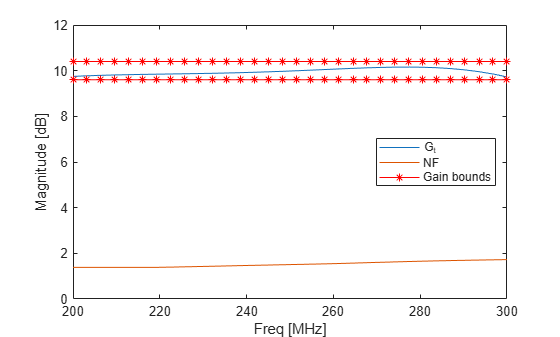``` 91 139 0 contract outside Optimization terminated: the current x satisfies the termination criteria using OPTIONS.TolX of 1.000000e-04 and F(X) satisfies the convergence criteria using OPTIONS.TolFun of 1.000000e-02 ```

### Update Matching Network and Analyze LNA

Update the input and output matching networks with optimized element values, `LC_Optimized`.

```InputMatchingNW.Elements(1).Inductance = LC_Optimized(1); InputMatchingNW.Elements(2).Inductance = LC_Optimized(2); InputMatchingNW.Elements(4).Inductance = LC_Optimized(3); InputMatchingNW.Elements(3).Capacitance = LC_Optimized(4); OutputMatchingNW.Elements(1).Inductance = LC_Optimized(5); OutputMatchingNW.Elements(2).Inductance = LC_Optimized(6); OutputMatchingNW.Elements(4).Inductance = LC_Optimized(7); OutputMatchingNW.Elements(3).Capacitance = LC_Optimized(8);```

Analyze the S-parameters of input and output matching networks and then convert the S-parameter data into an `nport` object.

```s1 = sparameters(InputMatchingNW,freq); np1 = nport(s1); s2 = sparameters(OutputMatchingNW,freq); np2 = nport(s2);```

Use the `rfbudget` object to calculate the transducer gain and the noise figure across the bandwidth. Compute RF budget of the LNA network. The LNA network comprises of matching network and low noise amplifier.

`bMatched = rfbudget([np1 clone(Mismatched_Amp) np2],freq',-30,BW);`

### Verify Design

Plot the transducer gain and the noise figure and then compare the optimization results with the mismatched network.

```plot(freq*1e-6,bMatched.TransducerGain(:,end)) hold all plot(freq*1e-6,bMismatched.TransducerGain(:,end)) plot(freq*1e-6,bMatched.NF(:,end)) plot(freq*1e-6,bMismatched.NF(:,end)) legend('G_t - Matched','G_t - Mismatched','NF - Matched',... 'NF - Mismatched','Location','East') xlabel('Frequency [MHz]') ylabel('Magnitude [dB]') axis([freq(1)*1e-6 freq(end)*1e-6 0 12]) grid on hold off```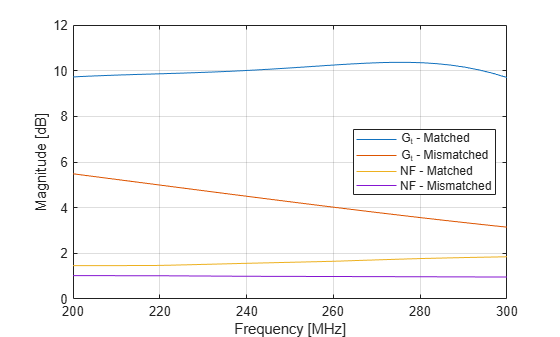The plot shows that the target requirement for both gain and noise figure have been met. To understand the effect of optimizing with respect to only the transducer gain, use the first choice for the cost function. The cost function involves only the gain term within the objective function.

### Display Optimized Element Values

Display the optimized inductor and capacitor values for the input matching network.

`Lin_Optimized = LC_Optimized(1:3)`
```Lin_Optimized = 3×1 10-7 × 0.5722 0.9272 0.3546 ```
`Cin_Optimized = LC_Optimized(4)`
```Cin_Optimized = 6.8526e-12 ```

Display the optimized inductor and capacitor values for the output matching network

`Lout_Optimized = LC_Optimized(5:7)`
```Lout_Optimized = 3×1 10-6 × 0.0517 0.1275 0.0581 ```
`Cout_Optimized = LC_Optimized(8)`
```Cout_Optimized = 5.4408e-12 ```

### Matching Network with Real-world Lumped Component Models

The real-world lumped components are selected from the Modelithics Select+ Library . You must have the Modelithics Select+ Library license to run the following codes. Note that the Modelithics® LC elements are scaled with respect to the substrate characteristics. This example shows one possible approach using a 59 mil FR4 substrate.

### Create RF Component from Modelithics SELECT+ Library

Set up the Modelithics Select+ Library by specifying the full path to the library.

```mdlxSetup('C:\mdlx_library\SELECT') ```

Create the Modelithics® library object.

```mdlx = mdlxLibrary; ```

Search the library for inductors with the optimized value for the input matching network.

```search(mdlx,'FR4Standard59mil',Type='Inductors',Value=Lin_Optimized(1)) ```Search the library for capacitors with the optimized value for the input matching network.

```search(mdlx,'FR4Standard59mil',Type='Capacitors',Value=Cin_Optimized) ```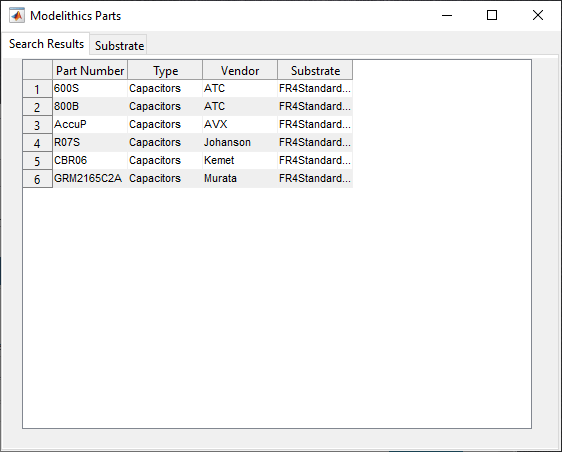Create an array of Modelithics® LC elements for the input and output matching networks.

```Lin1_mdlx = search(mdlx,'FR4Standard59mil',Type='Inductors',Value=Lin_Optimized(1)); Lin2_mdlx = search(mdlx,'FR4Standard59mil',Type='Inductors',Value=Lin_Optimized(2)); Lin3_mdlx = search(mdlx,'FR4Standard59mil',Type='Inductors',Value=Lin_Optimized(3)); Cin_mdlx = search(mdlx,'FR4Standard59mil',Type='Capacitors',Value=Cin_Optimized); Lout1_mdlx = search(mdlx,'FR4Standard59mil',Type='Inductors',Value=Lout_Optimized(1)); Lout2_mdlx = search(mdlx,'FR4Standard59mil',Type='Inductors',Value=Lout_Optimized(2)); Lout3_mdlx = search(mdlx,'FR4Standard59mil',Type='Inductors',Value=Lout_Optimized(3)); Cout_mdlx = search(mdlx,'FR4Standard59mil',Type='Capacitors',Value=Cout_Optimized); ```

### Create and Anlyze Matching Networks

Create the input and output matching networks with Modelithics® capacitors and inductors. Note that most of the Modelithics® lumped components have two ports. Use the `setports` function to define the circuit as a 2-port network.

```InputMatchingNWMdlx = circuit; add(InputMatchingNWMdlx,[1 2 0 0],Lin1_mdlx(1)); add(InputMatchingNWMdlx,[2 0 0 0],Lin2_mdlx(1)); add(InputMatchingNWMdlx,[2 0 0 0],Cin_mdlx(1)); add(InputMatchingNWMdlx,[2 3 0 0],Lin3_mdlx(1)); setports(InputMatchingNWMdlx,[1 0],[3 0]); OutputMatchingNWMdlx = circuit; add(OutputMatchingNWMdlx,[1 2 0 0],Lout1_mdlx(1)); add(OutputMatchingNWMdlx,[2 0 0 0],Lout2_mdlx(1)); add(OutputMatchingNWMdlx,[2 0 0 0],Cout_mdlx(1)); add(OutputMatchingNWMdlx,[2 3 0 0],Lout3_mdlx(1)); setports(OutputMatchingNWMdlx,[1 0],[3 0]); ```

Analyze the S-parameters of input and output matching networks and then convert the S-parameter data into an `nport` object.

```sm1 = sparameters(InputMatchingNWMdlx,freq); npm1 = nport(sm1); sm2 = sparameters(OutputMatchingNWMdlx,freq); npm2 = nport(sm2); ```

Use the `rfbudget` object to calculate the transducer gain and the noise figure across the bandwidth. Compute RF budget of the LNA network. The LNA network comprises of matching network and low noise amplifier.

```bMatchedMdlx = rfbudget([npm1 clone(Mismatched_Amp) npm2],freq',-30,BW); ```

### Verify Design

Plot the transducer gain and the noise figure of the real-world matching network and then compare the optimization results with the mismatched network.

```figure plot(freq*1e-6,bMatchedMdlx.TransducerGain(:,end)) hold all plot(freq*1e-6,bMatched.TransducerGain(:,end)) plot(freq*1e-6,bMismatched.TransducerGain(:,end)) plot(freq*1e-6,bMatchedMdlx.NF(:,end)) plot(freq*1e-6,bMatched.NF(:,end)) plot(freq*1e-6,bMismatched.NF(:,end)) axis([freq(1)*1e-6 freq(end)*1e-6 0 12]) legend('G_t - Matched w/ Modelithics®','G_t - Matched w/ ideal',... 'G_t - Mismatched','NF - Matched w/ Modelithics®','NF - Matched w/ ideal',... 'NF - Mismatched','Position', [0.22643,0.47762,0.49643,0.25119]) xlabel('Frequency [MHz]') ylabel('Magnitude [dB]') grid on hold off ```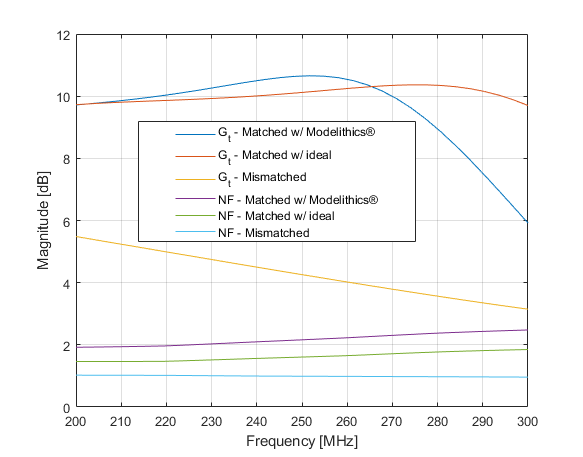The most noticeable aspect of the matching performance when using the Modelithics® library is the large deviation in transducer gain especially beyond 275 MHz. The noise figure performance has also worsened by approximately 0.25 dB. This can be explained by the fact that the inductor and capacitor models now represent real-world parasitic effects such as those due to the presence of a substrate, pads and orientation. This analysis highlights the importance of being able to predict such behaviors prior to realization of the design in hardware.

### References

 Ludwig, Reinhold, and Gene Bogdanov. RF Circuit Design: Theory and Applications. Upper Saddle River, NJ: Prentice-Hall, 2009.

 Cuthbert, Thomas R. Broadband Direct-Coupled and Matching RF Networks. Greenwood, Ark.: T.R. Cuthbert, 1999.

 Cuthbert, T.R. “A Real Frequency Technique Optimizing Broadband Equalizer Elements.” In 2000 IEEE International Symposium on Circuits and Systems. Emerging Technologies for the 21st Century. Proceedings (IEEE Cat No.00CH36353), 5:401–4. Geneva, Switzerland: Presses Polytech. Univ. Romandes, 2000. https://doi.org/10.1109/ISCAS.2000.857453.

 Pozar, David M. Microwave Engineering. 4th ed. Hoboken, NJ: Wiley, 2012.